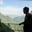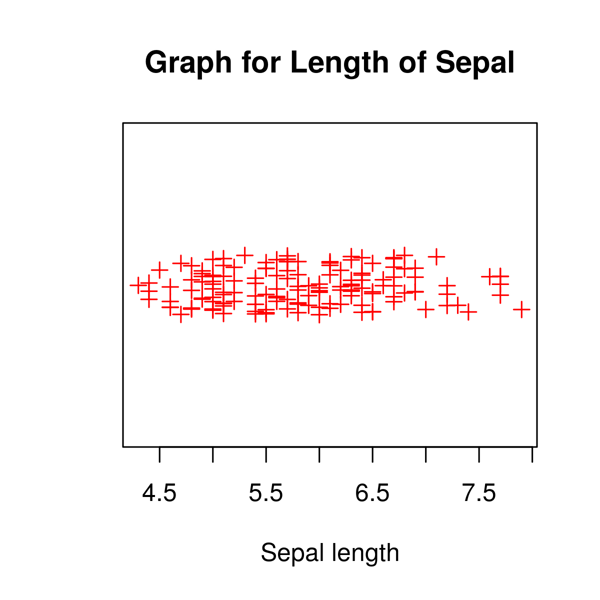Related Tags

r
communitycreator

# What are strip charts in R?AKASH BAJWA

In R programming, the stripchart() method is used to create strip charts. This method accepts a numeric vector and a list of more than one vector, then generates the strip chart for every vector.

### Syntax

stripchart(x, method, jitter, main, xlab, ylab, col, pch, vertical, group.names)


### Parameter

• x: It represents the numeric vector that has to be plotted. It may also contain the list of the vector.

• method: It helps to separate the points that have the same values. The method has an over-plot value to over-plot points by default. But it also has the option of jitter and stack.

• Jitter: This helps represent the amount of jittering that has to be applied.

• main: This shows the title of the chart.

• xlab: The label regarding x-axis.

• ylab: The label regarding y-axis.

• col: The color of the points regarding the plot.

• pch: The shape related to the points of the plot.

• vertical: The default dimension of the plot is horizontal. But if the vertical is set as true then the plot will be vertical.

• group.names: These are the group labels.

### Example

We are using a built-in mtcars dataset to generate the strip chart. data("mtcars") is used to load the pre-loaded dataset. In line 4, we generate a strip chart with multiple attributes (mpg, hp, wt, vs, gear etc.) in different ranges along the x-axis.

# 1. Load dataset(Pre-loaded)
data("mtcars")
# 2. Generate Strip Chart
stripchart(mtcars)
Expected Output

Now let’s import the iris dataset in our program and try to plot the strip chart using _jitter_.

By using:

• xlab= "Sepal length",

• method= "jitter",

• col= "Red",

• pch= 3,

• and the graph name "Graph for Length of Sepal",

we will get the following output.

# Load dataset
data("iris")
# Plotting chart
stripchart(iris\$Sepal.Length,
main="Graph for Length of Sepal",
xlab="Sepal length",
method="jitter",
col="Red",
pch=3
)Expected Output

RELATED TAGS

r
communitycreator

CONTRIBUTORAKASH BAJWA
RELATED COURSES

View all Courses

Keep Exploring

Learn in-demand tech skills in half the time